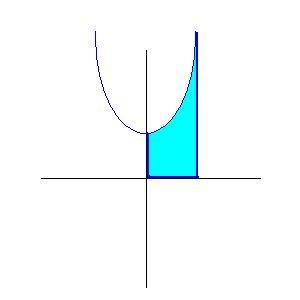# Solid of Revolution Question

• Piefrenzy

## Homework Statement

Find the volume of the solid generated by revolving the described region about the given axis:

The region enclosed above by the curve https://webwork.math.uga.edu/webwork2_files/tmp/equations/23/88cfb3fea3d8c8579f5a0608e8bd751.png, below by the https://webwork.math.uga.edu/webwork2_files/tmp/equations/fe/7ca85459d2390dbf4a5dfdd0b8b8e91.png-axis, to the left by the https://webwork.math.uga.edu/webwork2_files/tmp/equations/85/067ce783e2f89ced535d722b824af51.png-axis, and to the right by the line https://webwork.math.uga.edu/webwork2_files/tmp/equations/bd/6693b22701966f67dede0ee6737cf71.png, rotated about the https://webwork.math.uga.edu/webwork2_files/tmp/equations/85/067ce783e2f89ced535d722b824af51.png-axis.

## Homework Equations

We had been going over Volumes of Cylindrical Shells, so I'm pretty sure we need this formula

Integral from a to b = 2pi(shell radius)(shell height)dx

## The Attempt at a Solution

I made a nice graph of the equation, but I don't understand the constraints. Under the x-axis, to the left of the y-axis, and to the right of x = 2 makes no sense to me. Under the x-axis and to the left of the y-axis is Quadrant 3, but to the right of x = 2 is Q1 or Q2. I've been looking for examples in my book like this, but none of them are similar to this. I'm pretty sure once I understand the area it is talking about then I can do the rest myself.

## Homework Statement

Find the volume of the solid generated by revolving the described region about the given axis:

The region enclosed above by the curve https://webwork.math.uga.edu/webwork2_files/tmp/equations/23/88cfb3fea3d8c8579f5a0608e8bd751.png, below by the https://webwork.math.uga.edu/webwork2_files/tmp/equations/fe/7ca85459d2390dbf4a5dfdd0b8b8e91.png-axis, to the left by the https://webwork.math.uga.edu/webwork2_files/tmp/equations/85/067ce783e2f89ced535d722b824af51.png-axis, and to the right by the line https://webwork.math.uga.edu/webwork2_files/tmp/equations/bd/6693b22701966f67dede0ee6737cf71.png, rotated about the https://webwork.math.uga.edu/webwork2_files/tmp/equations/85/067ce783e2f89ced535d722b824af51.png-axis.
.

The area is bounded by the curve above, by the x-axis below, by the y-axis to the left and by x=2 to the right.The area is bounded by the curve above, by the x-axis below, by the y-axis to the left and by x=2 to the right.

View attachment 78283
Sometimes I wonder why they let me into college when I can't even read. I feel very dumb. I was reading " below the https://webwork.math.uga.edu/webwork2_files/tmp/equations/fe/7ca85459d2390dbf4a5dfdd0b8b8e91.png-axis" and so forth. Thank you very much, this is a lot easier now.

Sometimes I wonder why they let me into college when I can't even read. I feel very dumb. I was reading " below the https://webwork.math.uga.edu/webwork2_files/tmp/equations/fe/7ca85459d2390dbf4a5dfdd0b8b8e91.png-axis" and so forth. Thank you very much, this is a lot easier now.
It took me some time to understand, too. :)

It took me some time to understand, too. :)

Okay, so I made it to the very last problem with absolutely no issues, but for whatever reason I can't get this one right. Here it is:

Find the volume of the solid generated by revolving the described region about the given axis:

The region enclosed by the curve https://webwork.math.uga.edu/webwork2_files/tmp/equations/c2/8c66bf5b4a32e43b6a0dff6f0eb3ec1.png, and the lines https://webwork.math.uga.edu/webwork2_files/tmp/equations/c0/cbe04ad7371f4ea57957801971d85f1.png and https://webwork.math.uga.edu/webwork2_files/tmp/equations/ea/77a01c264d6993e42878959ebacc741.png rotated about the https://webwork.math.uga.edu/webwork2_files/tmp/equations/fe/7ca85459d2390dbf4a5dfdd0b8b8e91.png-axis.

I drew a graph of it, then saw it was around the x-axis, so I made everything in terms of y instead of x. My issue is usually the shell radius is just y, but in this case it changes later on (at x = 2 to be specific). I'm a little stuck. So right now I have: V = 2Π(y)(y2-(y+2))

However, I know this is definitely not correct. How exactly do I set this up?

I drew a graph of it, then saw it was around the x-axis, so I made everything in terms of y instead of x. My issue is usually the shell radius is just y, but in this case it changes later on (at x = 2 to be specific). I'm a little stuck. So right now I have: V = 2Π(y)(y2-(y+2))

However, I know this is definitely not correct. How exactly do I set this up?
I suggest the easiest way is to treat it as the difference of two volumes. Can you see from your picture what they are?
But it's not too bad your way either. First thing is to get the subtraction right. Which is greater here, y2 or y+2?

I suggest the easiest way is to treat it as the difference of two volumes. Can you see from your picture what they are?
But it's not too bad your way either. First thing is to get the subtraction right. Which is greater here, y2 or y+2?

Uh well I tried doing the integral of V1-V2 where V1 = 2Π(y)(y2) and V2 = 2Π(y)(y+2) and I get the same answer as V = 2Π(y)(y2-(y+2)) and the answer is still wrong.

I'm pretty sure y2 is greater than y+2, because otherwise I get a negative value.

just realized you are writing ##V = 2\pi y(y^2-(y+2))##. Don't you mean ##V = \int 2\pi y(y^2-(y+2)).dy##?
In the region, 0< y<2, so y+2>y2.

My issue is usually the shell radius is just y, but in this case it changes later on (at x = 2 to be specific). I'm a little stuck. So right now I have: V = 2Π(y)(y2-(y+2))

However, I know this is definitely not correct. How exactly do I set this up?

You have to get a number for the volume, not a function of y. Show your work in detail.